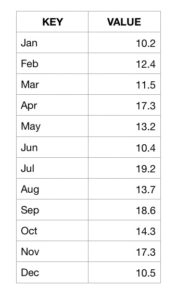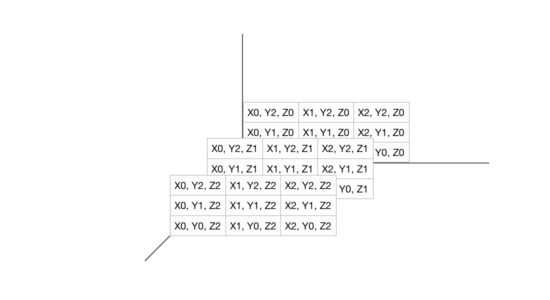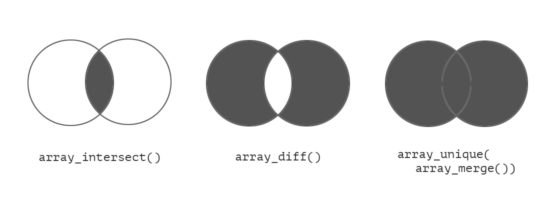# PHP Arrays

In PHP, array is an implementation of an ordered Map. A map is an abstract data type of key value pairs. It is an interface, a contract.

A map can have different implementations like a HashTable, HashMap, Dictionary, etc. In PHP, underlying implementation of an array actually points to a HashTable.

So if you are looking for a HashTable data type in PHP, look nowhere, it is the Array itself.

To know the internals, I recommend you to go through the PHP source for Array and HashTable.

You can use the array to store a group of data and refer them via a single variable name. Each array item is stored as a key and value pair.

Arrays are classified as “indexed array” and “associative array” based on the key specification. The Indexed array has default index that starts with ‘0’. The associative array contains the user-defined key index.

Keys are not restricted to natural numbers. You can use strings as well.## What is inside?

1. How to create an Array in PHP?
2. How to find the size of a PHP Array?
3. How to convert an array to a string in PHP?
4. How to convert a string to an array in PHP?
5. Finding intersection, difference and union of arrays
6. Creating variables from array keys
7. Frequently used built-in Array functions

## How to create an Array in PHP?

### An empty array

``````<?php
\$animals = array(
"Lion",
"Tiger",
"Wolf"
);
\$arrLength = count(\$animals);

// loop through the array
for (\$i = 0; \$i < \$arrLength; \$i ++) {
echo \$animals[\$i];
echo "</ br>";
}
?>
``````

### Associative array

``````<?php
\$animals = array(
"Lion" => "Wild",
"Sheep" => "Domestic",
"Tiger" => "Wild"
);

// loop through associative array and get key value pairs
foreach (\$animals as \$key => \$value) {
echo "Key=" . \$key . ", Value=" . \$value;
echo "</br>";
}
?>
``````

### Two dimensional array

``````<?php

// two-dimensional array definition
\$animals = array
(
array("Lion","Wild",8),
array("Sheep","Domestic",12),
array("Tiger","Wild",20)
);

// two-dimensional array iteration
for (\$row = 0; \$row < 3; \$row++) {
echo "<p>Row number \$row</p>";
echo "<ul>";
for (\$col = 0; \$col < 3; \$col++) {
echo "<li>".\$animals[\$row][\$col]."</li>";
}
echo "</ul>";
}
?>
``````

### Via loop

``````<?php
\$animals = array();
for (\$i = 0; \$i < \$count; \$i ++) {
\$animals[\$i] = array(
\$animalName[\$i],
\$animalType[\$i]
);
}
?>
``````

### Three dimensional array``````<?php
\$threeDArray = array(
array(
array(
"Lion",
"Tiger"
),
array(
"Sheep",
"Dog"
)
),
array(
array(
"Apple",
"Orange"
),
array(
"Tomato",
"Potato"
)
)
);
?>
``````

## How to find the size of a PHP Array?

You can use `count` function to find the size of an array. `sizeof` is an alias of the function `count`.

You might think what is the big deal with count function. If you are a beginner, you will definitely learn something new, so read on and do not skip this. Also remember to check the example script.

### count

The syntax of PHP `count` function is,

``````count(var array_variable, mode)
``````

If the value of `array_variable` is not set or having an empty array, then `count` will return 0. And, if it is not an array, then count will return 1. In PHP version 7 onwards, you will get a warning.

So whenever you need to check if a PHP array is empty, you need to use the count function. Ensure that you pass array to the count function.

``````<?php
if (count(\$array) > 0) {
echo "array has elements";
} else {
echo "array is empty";
}
?>
``````

The argument `mode` is optional and it specifies the mode of operation to be used in calculating array length. Possible options are,

• COUNT_NORMAL – will return the length of an array in depth 0. This is the default.
• COUNT_RECURSIVE – for recursive count operation, till it reaches depth n. This recursive call will be continued infinitely, till it reaches depth n or the program execution time limit is elapsed.

#### Example: PHP count() with mode option

Following script is an example for `count` function to calculate a number of elements available to a depth level. Let us use a two-dimensional array so that we can specify the mode depth.

``````<?php
\$toys = array(
array(
"name" => "Mechanical Cars",
"category" => "pull back"
),
array(
"name" => "Jigsaw",
"category" => "puzzles"
),
array(
"name" => "HiTech Cars",
"category" => "remote"
),
array(
"name" => "Teddy Bears",
"category" => "soft"
),
array(
"name" => "Baby pillow",
"category" => "soft"
),
array(
"name" => "Chinese Checker",
"category" => "puzzles"
),
array(
"name" => "Jumbo Helicopter",
"category" => "remote"
)
);
?>
``````

Now, we can invoke `count` function by passing different possible `mode` options.

``````<?php
\$array_length["normal_count"] = count(\$toys, COUNT_NORMAL);
// (OR)
\$array_length["default_count"] = count(\$toys);
\$array_length["recursive_count"] = count(\$toys, COUNT_RECURSIVE);
print "<pre>";
print_r(\$array_length);
print "</pre>";
?>
``````

In the above PHP code, the count() is invoked with normal, default and recursive mode. Returned values are stored into an array named, \$array_length, by specifying the appropriate name indices.

Following is the output of this program.

``````Array
(
[normal_count] => 7
[default_count] => 7
[recursive_count] => 21
)
``````

### sizeof

This function `sizeof` is an alias of PHP `count` function. It accepts the same set of arguments as like as `count()`.

We can replace the `count` with `sizeof` in the above PHP code to compare the results of both functions which will be same for both functions calls.

Alias functions in PHP are preserved for providing backward compatibility. If you are writing a new program it is better not to go with the alias functions as there is a chance to deprecate it in the future.

## How to convert an array to a string in PHP?

You can use the built-in function `implode` to convert an array to a string.

### PHP Implode

`implode` function accepts an array and a separator as parameters. It combines each of the array elements and separates them with the passed separator argument. For example,

``````<?php
\$str_concat = "|";
\$meaning_array = array(
"implode",
"join",
"concatenate"
);
\$imploded_string = implode(\$str_concat, \$meaning_array);
echo \$imploded_string; // implode|join|concatenate
?>
``````

If you use a comma (,) as separator, then you will be able to covert the array as CSV. This will be a useful utility in many situations.

## How to convert a string to an array in PHP?

You can use the built-in function explode to convert a string to an array. `split` function is deprecated and so do not use it for creating an array based on a string.

### PHP Explode

`explode` is to split the string into an array of elements based on a given separator. explode accepts separator and a string as its argument.

``````<?php
\$input_string = "implode|join|concatenate";
\$str_explode = "|";
\$exploded_array = explode(\$str_explode, \$input_string);
echo "<pre>";
print_r(\$exploded_array);
echo "</pre>";
?>
``````

This example code accepts the resultant string data of implode example we just saw above. Explode splits string data based on the argument separator “|”. The output is,

``````Array
(    => implode
 => join
 => concatenate
)
``````

#### Explode within Limit

PHP explode() accepts a third optional argument `limit` to set target length or boundary for performing explode operation. `explode` will take entire string as default if `limit` is not specified.

``````<?php
\$exploded_array = explode(\$str_explode, \$input_string, 2);
?>
``````

this will return,

``````Array
(    => implode
 => join|concatenate
)
``````

## Finding intersection, difference and union of arrays

PHP has wide array of in-built functions for handling arrays. Intersection, difference and union are performed quite regularly when using arrays. PHP has got good set of functions in-built to handle these operations.### Array intersection

PHP array_intersect function is used to find intersection between arrays. This function can accept multiple arguments to compare. After comparison, this function returns an array of intersecting elements that are present in all the argument arrays.

Syntax:

``````<?php
array_intersect(\$input_array1, \$input_array2, \$input_array3);
?>
``````

#### Array intersection example

In this example, we are going to pass four arrays as parameters.

``````<?php
\$soft_toys = array(
"Baby Pillow",
"Teddy Bear",
"Chicklings"
);
\$baby_toys = array(
"Toy phone",
"Baby Pillow",
"Lighting Ball",
"Teddy Bear"
);
"Coloring Fun",
"Baby Pillow",
"Barbies",
"Teddy Bear"
);
\$kids_bedroom_set = array(
"Teddy Bear",
"Baby Pillow",
"Night Lamp",
"Kids Bed"
);
\$arrayIntersect = array_intersect(\$soft_toys, \$baby_toys, \$gift_items, \$kids_bedroom_set);
print "<PRE>";
print_r(\$arrayIntersect);
print "</PRE>";
?>
``````

This program prints resultant array containing values that are present in all the four input arrays. The output is,

``````Array(
 => Baby Pillow
 => Teddy Bear
)
``````

#### Other Array intersection functions in PHP

PHP includes more variety of functions to calculate intersection of elements among input arrays.

• `array_intersect_key` – returns intersecting elements with respect to the keys.
• `array_interset_assoc` – returns intersecting elements with respect to keys and values.
• `array_intersect_ukey` – returns intersecting elements on the basis of custom function by providing an algorithm for the intersection.

### Array difference

By using PHP array difference functions, we can find differences between arrays with respect to their keys or values.

Array differences can also be found, based on the index check by using the custom made functions. They are used as a callback.

• array_diff_ukey
• array_diff_uassoc

The list goes on. By comparing their entries with the use of user-defined callback functions.

• array_udiff
• array_udiff_assoc
• array_udiff_uassoc

#### array_diff

It accepts at least two arrays, but, maximum in any number of arrays, as its arguments, thereby, the elements from the first array will be compared with the rest of the array elements.

#### array_diff_key

This function is used as similar as array_diff, but for comparing array elements with respect to their key.

#### array_diff_assoc

This function is used to compare the values between given arrays, by considering the key index associated with these values.

#### Array difference example

``````<?php
\$array_from = array(
"fruit1" => "apple",
"fruit2" => "grapes",
"friut3" => "banana"
);
\$array_against = array(
"fruit1" => "orange",
"fruit2" => "grapes",
"friut4" => "banana"
);
\$value_differences = array_diff(\$array_from, \$array_against);
\$key_differences = array_diff_key(\$array_from, \$array_against);
\$assoc_index_differences = array_diff_assoc(\$array_from, \$array_against);
print "<PRE>";
print_r(\$value_differences);
print_r(\$key_differences);
print_r(\$assoc_index_differences);
print "</PRE>";
?>
``````

Array difference example’s output below:

``````Array
(
[fruit1] => apple
)
Array
(
[friut3] => banana
)
Array
(
[fruit1] => apple
[friut3] => banana
)
``````

#### PHP Array difference with callback – closures

It is possible to define your own custom logic to find difference between arrays. User-defined functions can be passed for callback.

Passing functions as argument is a cool feature in PHP. They are called anonymous functions and implemented using closures.

• array_diff_ukey , array_diff_uassoc for key and index based differences.
• array_udiff , array_udiff_assoc for value based array difference.
• array_udiff_uassoc used to perform both array value and key index comparison.

## Creating variables from array keys

PHP has a function named extract to create variables using array keys. It creates PHP variables with the name of each array keys. It uses an associative array as input argument.

### extract

This function accepts three arguments as listed below.

• \$input_array – Associative array from which the keys are converted into PHP variables.
• \$extract_type – Defines options to create a new variable or overwrite existing variables.
• EXTR_OVERWRITE, EXTR_SKIP, EXTR_IF_EXISTS, EXTR_REFS are the options.
• \$variable_prefix – String to prefix with the variable name to be created.

#### PHP extract example

``````<?php
\$partA = "";
\$partB = "";
\$input_array = array(
"partA" => "Stories",
"partB" => "Rhymes",
"partC" => "Concepts"
);
extract(\$input_array, EXTR_OVERWRITE);
print '\$partA=' . \$partA . '<br/>';
print '\$partB=' . \$partB . '<br/>';
print '\$partC=' . \$partC . '<br/>';
?>
``````

#### Caution on using extract

You should not use extract on user input data. If you do so, it may cause security concerns. Do not use this function, unless otherwise you know what you are doing!

## Frequently used built-in Array functions

Let us see some of the widely used PHP array functions with PHP code snippet examples.

### range

PHP `range()` function is used get the array of elements in the range between the start and end parameters. The range can be a numeric or alphabet. The following code shows how to get the array of alphabets that exists between “e” and “i”.

``````<?php
\$character_array = range('e', 'i'); // returns array('e','f','g','h','i');
?>
``````

### reset

The `reset()` function is used to reset the position of the array pointer. It requires the array variable as its argument, to move the array pointer to its original position.

``````<?php
\$character_array = range('e', 'i'); // returns array('e','f','g','h','i');
print next(\$character_array); // prints f
print next(\$character_array); // prints g
reset(\$character_array);
print current(\$character_array); // prints the first element 'e' after reset
?>
``````

### array_slice

It is used to get the sub-array from the given input array. It accepts the input array, starting position as offset and the length of the sub array.

The length parameter is optional. If it is not specified, the length of the input array will be taken by default. The fourth argument is to preserve keys by avoiding the default index reordering behavior.

``````<?php
\$color_array = array(
"Red",
"Blue",
"Green",
"Yellow",
"Brown"
);
\$sliced_array = array_slice(\$color_array, 1, 3); // now the sliced array will be array("Blue","Green","Yellow")
?>
``````

### array_splice

This function is similar to array_slice and it can also replace the removed elements with the new set of elements. The length of the removed set and new array should be same.

``````<?php
\$character_array = array(
'e',
'f',
'g',
'h',
'i'
);
\$number_array = array(
'1',
'2',
'3'
);
\$changed_array = array_splice(\$character_array, 1, 3, \$number_array); // will hold the element like: array('e','1','2','3','i');
?>
``````

### shuffle

This function has the array as an argument to change the position of its value in a random manner.

### array_chunk

`array_chunk()` is used to break an array into the specified length of chunks.

``````<?php
\$character_array = array(
'e',
'f',
'g',
'h',
'i'
);
\$chunks = array_chunk(\$character_array, 2);
// \$chunks will be as follows
/*
* Array (
*  => Array (
*  => e
*  => f
* )
*  => Array (
*  => g
*  => h
* )
*  => Array (
*  => i
* )
* )
*/
?>
``````

### merge

`merge` function, is for joining one or more arrays into a single array.

``````<?php
\$color_array1 = array(
"Red",
"Blue",
"Green"
);
\$color_array2 = array(
"Yellow",
"Brown"
);
// returns array("Red","Blue","Green","Yellow","Brown");
\$color_array = array_merge(\$color_array1, \$color_array2);
?>
``````

### array_key_exists

This function is used to check whether the given key exists in the array or not. This function takes a key element and the input array as arguments.

### array_keys, array_values

These two functions will return the keys and values of an input array, respectively. The following code shows the usage method of these functions and the resultant array of keys and values.

``````<?php
\$student_array = array(
"name" => "Martin",
"mark" => "90",
);
\$key_array = array_keys(\$student_array); // returns array("name","mark","grade")
\$value_array = array_values(\$student_array); // returns array("Martin","90","A")
?>
``````

### array_push, array_pop, array_shift, array_unshift

`array_push()` is used to push values into an array. Using this function, a new element will be added to the end of a given input array.

The `array_pop()` is used to get the last element of the array.

The `array_shift()` and the `array_unshift()` methods are as similar as `array_push()` and `array_pop()`But, the insert and retrieve operations are performed at the starting position of the array.

### extract, compact

The `compact()` is used to convert the variable into an array, whereas, the `extract()` is used to extract an array and convert the element of that array as variables.

The example shows it as follows. The second and third argument of extract() is optional. It is used to add a prefix to the variable name.

``````<?php
\$student_array = array(
"name" => "Martin",
"mark" => "90",
);
extract(\$student_array, EXTR_PREFIX_ALL, "student");
echo \$student_name . "-" . \$student_mark . "(" . \$student_grade . ")"; // prints as Martin - 90(A)
\$compact_array = compact("student_name", "student_mark", "student_grade"); // will form an array
?>
``````

• Evan says:

I am learning PHP for work.
And starting with PHP 7 I see there is a renaissance of respect for the language.
But the logo for PHP is embarrasing (the oval).

If the favicon from your website were the logo for PHP I would be more proud to learn the language! Including the orange color.

• Vincy says:

Hi Evan,

It is a persona taste :-)

Best wishes for you to learn PHP.